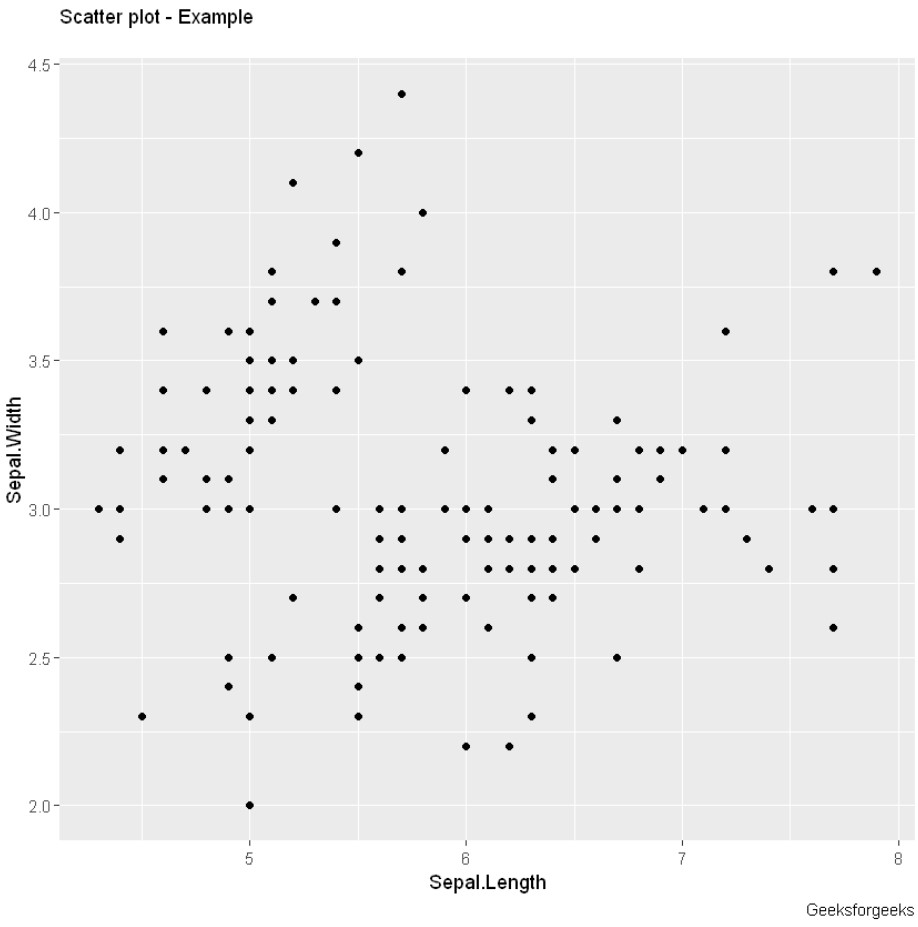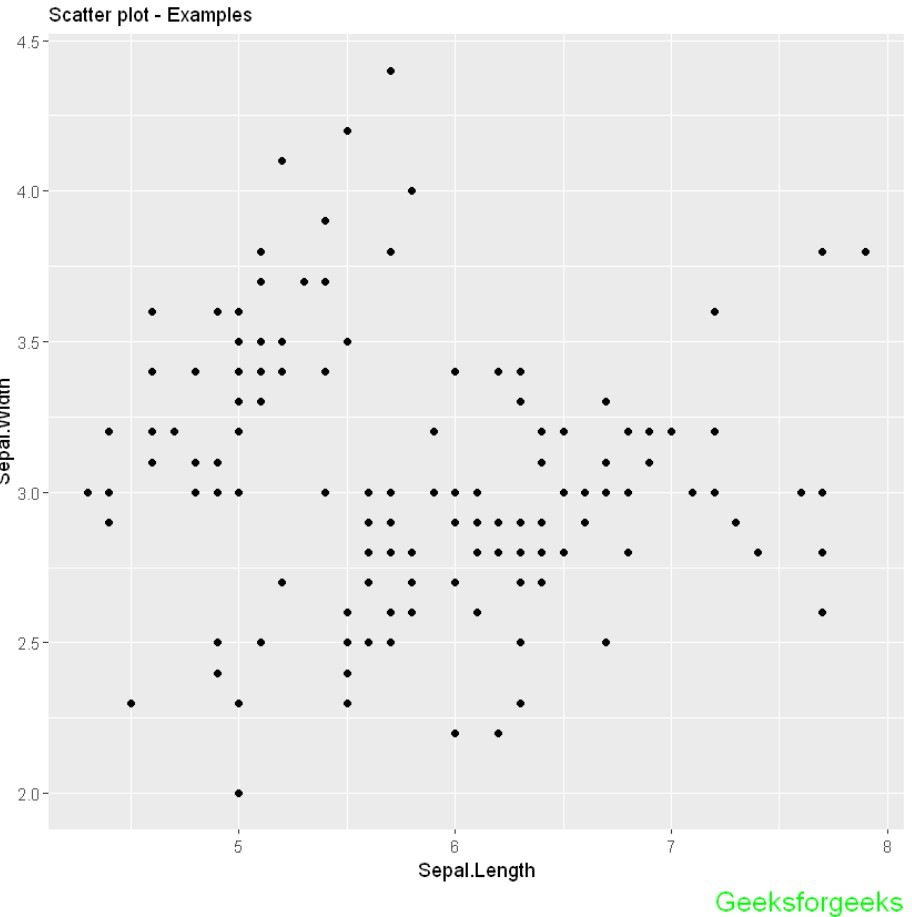# How to Add Caption to a ggplot in R?

• Last Updated : 27 Jan, 2022

In this article, we are going to see how we can add a caption to a plot in R Programming Language. The caption is much important in data visualization to display some details related to graphs.

## Preparing Data

To plot the scatterplot we will use we will be using the geom_point() function. Following is brief information about the ggplot function, geom_point().

Syntax : geom_point(size, color, fill, shape, stroke)

Parameter :

• size : Size of Points
• color : Color of Points/Border
• fill : Color of Points
• shape : Shape of Points in in range from 0 to 25
• stroke : Thickness of point border
• Return : It creates scatterplots.

## R

 `# import lib ` `library``(ggplot2) ` ` `  `# plot datapoint using iris ` `ggplot``(iris, ``aes``(x = Sepal.Length, y = Sepal.Width)) + ` `    ``geom_point``() `

Output:## Adding a caption to a plot

To add caption we will use caption attributes from labs() function.

Syntax: labs(caption)

Parameters:

• caption: String caption

## R

 `library``(ggplot2) ` `ggplot``(iris, ``aes``(x = Sepal.Length, y = Sepal.Width)) + ` `    ``geom_point``()+ ` ` `  `    ``# adding caption and subtitle ` `    ``labs``(subtitle=``"Scatter plot"``, ` `       ``caption=``"Geeksforgeeks"` `       ``)`

Output:## Customize caption text

element_text() methods can be used to customize the caption within graph

## R

 `library``(ggplot2) ` `ggplot``(iris, ``aes``(x = Sepal.Length, y = Sepal.Width)) + ` `    ``geom_point``()+ ` ` `  `    ``# adding caption and subtitle ` `    ``labs``(subtitle=``"Scatter plot - Examples"``, ` `       ``caption=``"Geeksforgeeks"``)+  ` ` `  `    ``# size of the caption ` `    ``theme``(plot.caption= ``element_text``(size=15, ` `                                   ``color=``"Green"``))`

Output:My Personal Notes arrow_drop_up
Recommended Articles
Page :